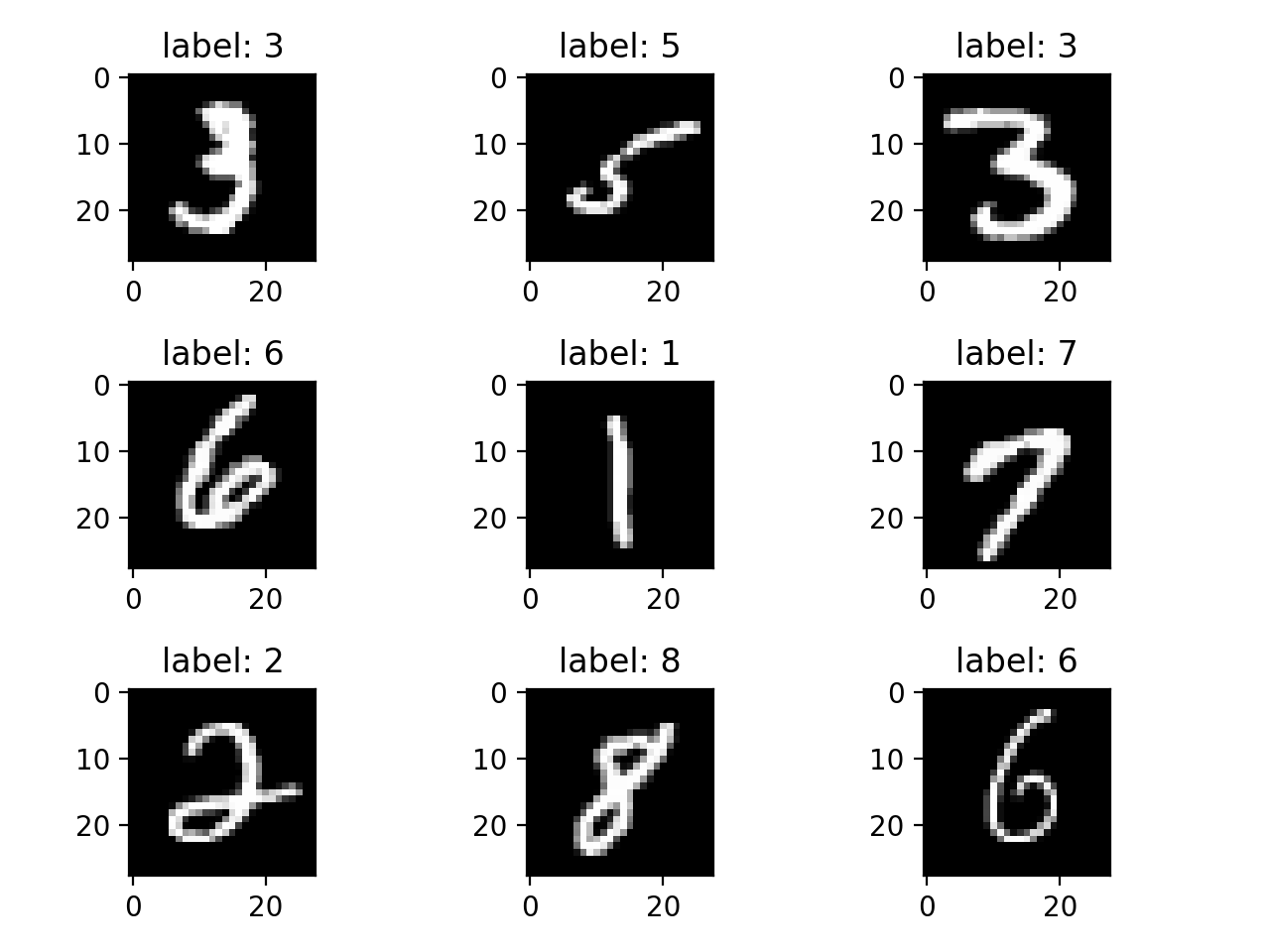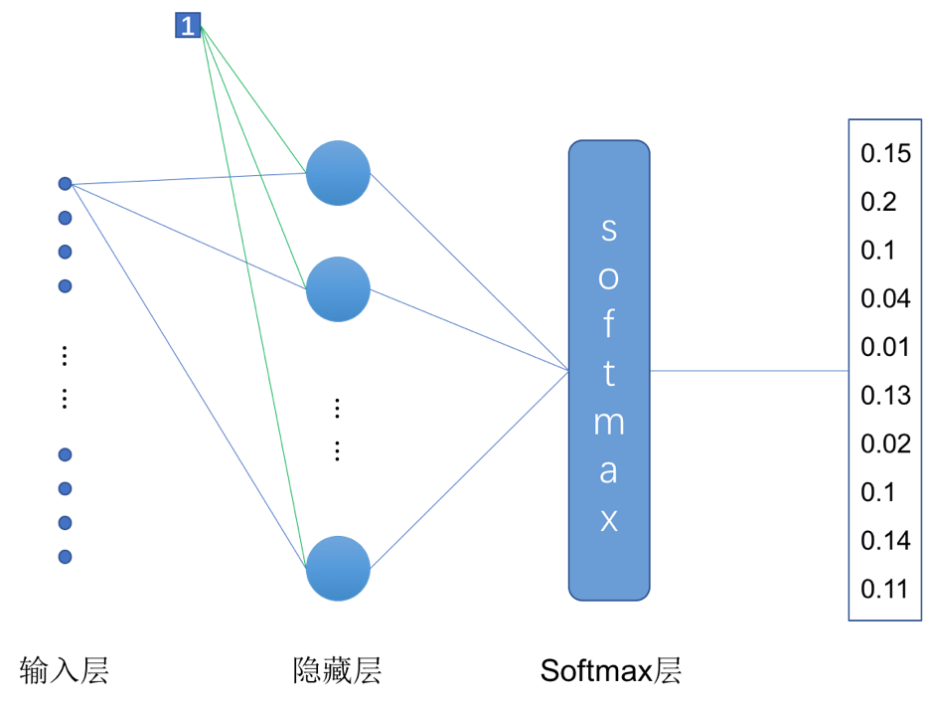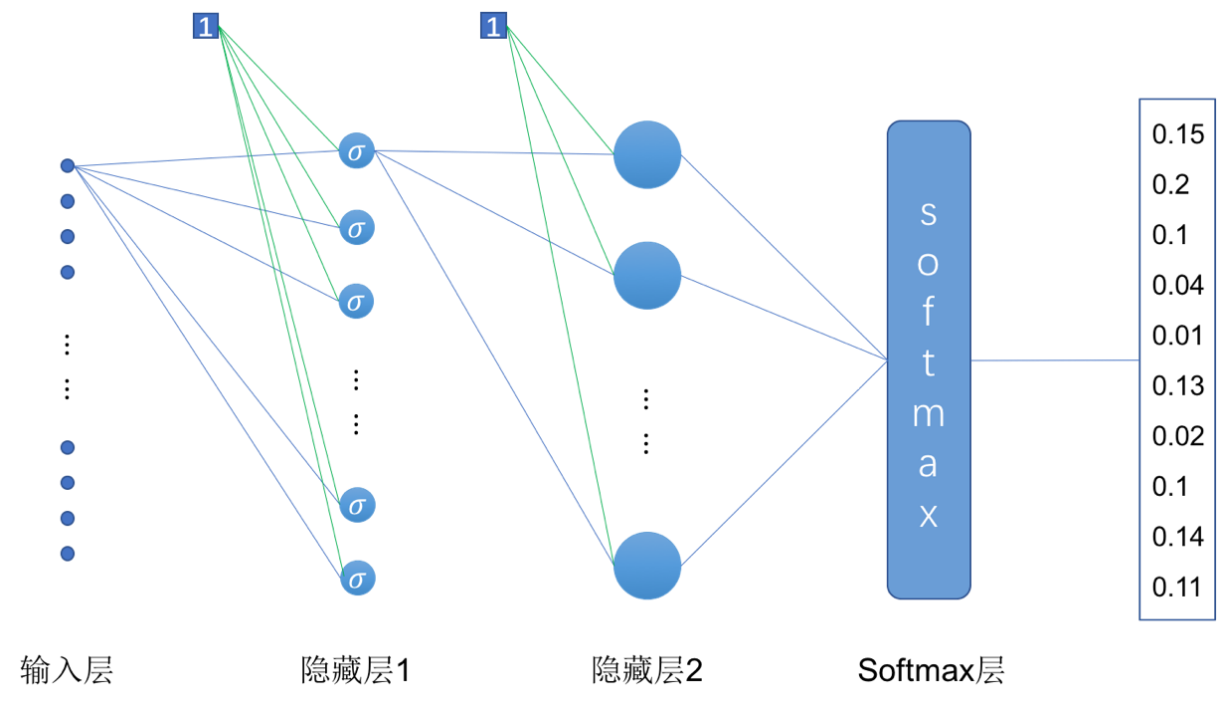# TensorFlow系列专题（六）：实战项目Mnist手写数据集识别

• 导读
• MNIST数据集
• 数据处理
• 单层隐藏层神经网络的实现
• 多层隐藏层神经网络的实现
• 导读

• MNIST数据集

MNIST数据集可以从网站http://yann.lecun.com/exdb/mnist/上下载，需要下载的数据集总共有4个文件，其中“train-images-idx3-ubyte.gz”是训练集的图片，总共有60000张，“train-labels-idx1-ubyte.gz”是训练集图片对应的类标（0~9）。“t10k-images-idx3-ubyte.gz”是测试集的图片，总共有10000张，“t10k-labels-idx1-ubyte.gz”是测试集图片对应的类标（0~9）。TensorFlow的示例代码中已经对MNIST数据集的处理进行了封装，但是作为第一个程序，我们希望带着读者从数据处理开始做，数据处理在整个机器学习项目中是很关键的一个环节，因此有必要在第一个项目中就让读者体会到它的重要性。

 [offset] [type] [value] [description] 0000 32 bit integer 0x00000803(2051) magic number 0004 32 bit integer 60000 number of images 0008 32 bit integer 28 number of rows 0012 32 bit integer 28 number of columns 0016 unsigned byte ?? pixel 017 unsigned byte ?? pixel …… xxxx unsigned byte ?? pixel

 [offset] [type] [value] [description] 0000 32 bit integer 0x00000801(2049) magic number(MSB first) 0004 32 bit integer 60000 number of items 0008 unsigned byte ?? label 0009 unsigned byte ?? label …… xxxx unsigned byte ?? label• 数据处理

 1 import numpy as np 2 import struct 3 import random 4 5 class MnistData: 6 def __init__(self, train_image_path, train_label_path, 7 test_image_path, test_label_path): 8 # 训练集和测试集的文件路径 9 self.train_image_path = train_image_path 10 self.train_label_path = train_label_path 11 self.test_image_path = test_image_path 12 self.test_label_path = test_label_path 13 14 # 获取训练集和测试集数据 15 # get_data()方法，参数为0获取训练集数据，参数为1获取测试集 16 self.train_images, self.train_labels = self.get_data(0) 17 self.test_images, self.test_labels = self.get_data(1) 18 19 # 定义两个辅助变量，用来判断一个回合的训练是否完成 20 self.num_of_batch = 0 21 self.got_batch = 0

 22 def get_data(self, data_type): 23 if data_type == 0:  # 获取训练集数据 24 image_path = self.train_image_path 25 label_path = self.train_label_path 26 else:  # 获取测试集数据 27 image_path = self.test_image_path 28 label_path = self.test_label_path 29 30 with open(image_path, ‘rb’) as file1: 31 image_file = file1.read() 32 with open(label_path, ‘rb’) as file2: 33 label_file = file2.read() 34 35 label_index = 0 36 image_index = 0 37 labels = [] 38 images = [] 39 40 # 读取训练集图像数据文件的文件信息 41 magic, num_of_datasets, rows, columns =\ 42 struct.unpack_from(‘>IIII’, image_file, image_index) 43 image_index += struct.calcsize(‘>IIII’) 44 45 for i in range(num_of_datasets): 46 # 读取784个unsigned byte，即一副图像的所有像素值 47 temp = struct.unpack_from(‘>784B’, image_file, image_index) 48 # 将读取的像素数据转换成28*28的矩阵 49 temp = np.reshape(temp, (28, 28)) 50 # 归一化处理 51 temp = temp / 255 52 images.append(temp) 53 image_index += struct.calcsize(‘>784B’)  # 每次增加784B 54 55 # 跳过描述信息 56 label_index += struct.calcsize(‘>II’) 57 labels = struct.unpack_from(‘>’ + str(num_of_datasets) 58 + ‘B’, label_file, label_index) 59 60 # one-hot 61 labels = np.eye(10)[np.array(labels)] 62 63 return images, labels

 def get_batch(self, batch_size): # 刚开始训练或当一轮训练结束之后，打乱数据集数据的顺序 if self.got_batch == self.num_of_batch: train_list = list(zip(self.train_images, self.train_labels)) random.shuffle(train_list) self.train_images, self.train_labels = zip(*train_list) # 重置两个辅助变量 self.num_of_batch = 60000 / batch_size self.got_batch = 0 # 获取一个batch size的训练数据 train_images = self.train_images[ self.got_batch*batch_size:(self.got_batch+1)*batch_size] train_labels = self.train_labels[ self.got_batch*batch_size:(self.got_batch+1)*batch_size] self.got_batch += 1 return train_images, train_labels1 from mnist_data import MnistData 2 import tensorflow as tf

 3 # 创建Session会话 4 sess = tf.InteractiveSession() 5 6 # 训练集、测试集的文件路径 7 train_image_path = ‘./data/train-images-idx3-ubyte’ 8 train_label_path = ‘./data/train-labels-idx1-ubyte’ 9 test_image_path = ‘./data/t10k-images-idx3-ubyte’ 10 test_label_path = ‘./data/t10k-labels-idx1-ubyte’ 11 12 epochs = 10          # 训练的总轮数 13 batch_size = 100      # 每个batch的大小 14 learning_rate = 0.2    # 学习率

“epochs”是我们想要训练的总轮数，每一轮都会使用训练集的所有数据去训练一遍模型。由于我们使用随机梯度下降方法更新参数，所以不会一次把所有的数据送进模型去训练，而是按批次训练，“batch_size”是我们定义的一个批次的数据量的大小，这里我们设定了100，那么每个“batch”就会送100个样本到模型中去训练，一轮训练的“batch”数等于总的训练集数量除以“batch_size”。“learning_rate”是我们定义的学习率，即模型参数更新的速率。

 15 # 创建样本数据的placeholder 16 x = tf.placeholder(tf.float32, [None, 28, 28]) 17 # 定义权重矩阵和偏置项 18 W = tf.Variable(tf.zeros([28*28, 10])) 19 b = tf.Variable(tf.zeros()) 20 21 # 样本的真实标签 22 y_ = tf.placeholder(tf.float32, [None, 10]) 23 # 使用softmax函数将单层网络的输出转换为预测结果 24 y = tf.nn.softmax(tf.matmul(tf.reshape(x, [-1, 28*28]), W) + b)

 # 损失函数和优化器 # -tf.reduce_sum(y_ * tf.log(y) 计算这个batch中每个样本的交叉熵 # reduce_mean方法对一个batch的样本的交叉熵求平均值，作为最终的loss cross_entropy = tf.reduce_mean(-tf.reduce_sum(y_ * tf.log(y), axis=1)) train_step = \ tf.train.GradientDescentOptimizer(learning_rate).minimize(cross_entropy)

 31 # 比较预测结果和真实类标 32 correct_prediction = tf.equal(tf.argmax(y, 1), tf.argmax(y_, 1)) 33 # 计算准确率 34 accuracy = tf.reduce_mean(tf.cast(correct_prediction, tf.float32))

“tf.equal()”方法用于比较两个矩阵或向量相应位置的元素是否相等，相等为“True”，不等为“False”。“tf.cast”用于将“True”和“False”转换为“1”和“0”，“tf.reduce_mean”对转换后的数据求平均值，该值即为模型在测试集上预测结果的准确率。最后，我们实现模型的训练和预测：

 35 # 初始化MnistData类 36 data = MnistData(train_image_path, train_label_path, 37 test_image_path, test_label_path) 38 # 初始化模型参数 39 init = tf.global_variables_initializer().run() 40 41 # 开始训练 42 for i in range(epochs): 43 for j in range(600): 44 # 获取一个batch的数据 45 batch_x, batch_y = data.get_batch(batch_size) 46 # 优化参数 47 train_step.run({x: batch_x, y_: batch_y}) 48 49 # 对测试集进行预测并计算准确率 50 print(accuracy.eval({x: data.test_images, y_: data.test_labels}))

• 多层神经网络的实现# 定义权重矩阵和偏置项 w_1 = tf.Variable(tf.truncated_normal([28*28, 200], stddev=0.1)) b_1 = tf.Variable(tf.zeros()) w_2 = tf.Variable(tf.truncated_normal([200, 10], stddev=0.1)) b_2 = tf.Variable(tf.zeros())

 # 定义一个两层神经网络模型 y_1 = tf.nn.sigmoid(tf.matmul(tf.reshape(x, [-1, 28*28]), w_1) + b_1) y = tf.nn.softmax(tf.matmul(y_1, w_2) + b_2)